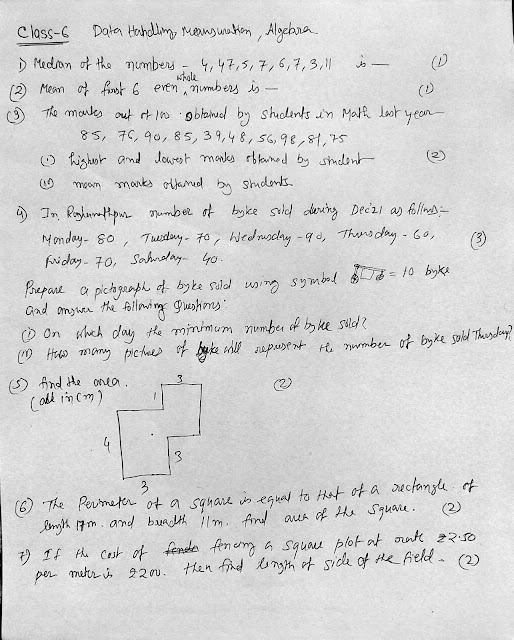28 Aug 2018

First True Mathematician-- Thales

Thales ( 624-546 BC) was an ancient Philosopher lived in Ancient Greece now forms a part of Turkey.
Thales regarded as the First True Mathematician , because of the many important contributions made to the field of Mathematics, Especially in Geometry.
Thales used geometry to calculate the distance of a ship from the shore. He also figured out a way to measure the height of a Pyramids.

Its a very interesting way. The time of day when his own shadow was same height that he was he measure the shadow of the Pyramid to get the height.
Thales was a great mathematician, He proves that:--

1. A circle is bisected or cut into halves by its diameter.
2. The angle at the base of an isosceles triangle are equal.
3. An angle is is the shape of the space between two straight lines at the point where they meet.
4. If two straight line cut one another, the opposite angles are equal. Now known as Vertically opposite angle.
5. If two triangles have two angle and a side in common, the triangles are identical. Now known as AAS (Angle Angle Side) Law.

1.Wao great

2.